JEE  >  Test: 35 Year JEE Previous Year Questions: Structure of Atom

# Test: 35 Year JEE Previous Year Questions: Structure of Atom

Test Description

## 29 Questions MCQ Test Chemistry for JEE | Test: 35 Year JEE Previous Year Questions: Structure of Atom

Test: 35 Year JEE Previous Year Questions: Structure of Atom for JEE 2023 is part of Chemistry for JEE preparation. The Test: 35 Year JEE Previous Year Questions: Structure of Atom questions and answers have been prepared according to the JEE exam syllabus.The Test: 35 Year JEE Previous Year Questions: Structure of Atom MCQs are made for JEE 2023 Exam. Find important definitions, questions, notes, meanings, examples, exercises, MCQs and online tests for Test: 35 Year JEE Previous Year Questions: Structure of Atom below.
Solutions of Test: 35 Year JEE Previous Year Questions: Structure of Atom questions in English are available as part of our Chemistry for JEE for JEE & Test: 35 Year JEE Previous Year Questions: Structure of Atom solutions in Hindi for Chemistry for JEE course. Download more important topics, notes, lectures and mock test series for JEE Exam by signing up for free. Attempt Test: 35 Year JEE Previous Year Questions: Structure of Atom | 29 questions in 60 minutes | Mock test for JEE preparation | Free important questions MCQ to study Chemistry for JEE for JEE Exam | Download free PDF with solutions
 1 Crore+ students have signed up on EduRev. Have you?
Test: 35 Year JEE Previous Year Questions: Structure of Atom - Question 1

### In a hydrogen atom, if energy of an electron in ground state is 13.6. ev, then that in the 2nd excited state is 

Detailed Solution for Test: 35 Year JEE Previous Year Questions: Structure of Atom - Question 1

2nd excited state will be the 3rd energy level.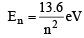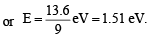Test: 35 Year JEE Previous Year Questions: Structure of Atom - Question 2

### Uncertainty in position of a minute particle of mass 25 g in space is 10–5 m. What is the uncertainty in its velocity (in ms–1) ? (h = 6.6 x 10-34 Js) 

Detailed Solution for Test: 35 Year JEE Previous Year Questions: Structure of Atom - Question 2

TIPS/Formulae :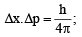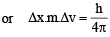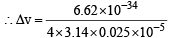= 2.1 x 10-28 ms-1

Test: 35 Year JEE Previous Year Questions: Structure of Atom - Question 3

### The number of d-electrons retained in Fe2+  (At. no. of Fe = 26) ion is

Detailed Solution for Test: 35 Year JEE Previous Year Questions: Structure of Atom - Question 3

Fe++ (26 – 2 = 24) = 1s2 2s2 2p6 3s2 3p6 4s0 3d6 hence no. of d electrons retained is 6. [Two 4s electron are removed]

Test: 35 Year JEE Previous Year Questions: Structure of Atom - Question 4

The orbital angular momentum for an electron revolving in an orbit is given by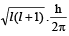This momentum for an s-electron will be given by 

Detailed Solution for Test: 35 Year JEE Previous Year Questions: Structure of Atom - Question 4

TIPS/Formulae : For s-electron, ℓ = 0

∴Orbital angular momentum =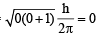Test: 35 Year JEE Previous Year Questions: Structure of Atom - Question 5

Which one of the following groupings represents a collection of isoelectronic species ?(At. nos. : Cs : 55, Br : 35)

Detailed Solution for Test: 35 Year JEE Previous Year Questions: Structure of Atom - Question 5

N3–, F and Na+ contain 10 electrons each.

Test: 35 Year JEE Previous Year Questions: Structure of Atom - Question 6

In Bohr series of lines of hydrogen spectrum, the third line from the red end corresponds to which one of the following inter-orbit jumps of the electron for Bohr orbits in an atom of hydrogen 

Planck’s constant, h = 6.63 × 10–34 Js

Detailed Solution for Test: 35 Year JEE Previous Year Questions: Structure of Atom - Question 6

The lines falling in the visible region comprise Balmer series. Hence the third line from red would be n1 =2,
n2 = 5 i.e. 5 → 2.

Test: 35 Year JEE Previous Year Questions: Structure of Atom - Question 7

The de Broglie wavelength of a tennis ball of mass 60 g moving with a velocity of 10 metres per second is approximately 

Detailed Solution for Test: 35 Year JEE Previous Year Questions: Structure of Atom - Question 7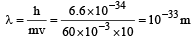Test: 35 Year JEE Previous Year Questions: Structure of Atom - Question 8

Which of the following sets of quantum numbers is correct for an electron in 4f orbital ? 

Detailed Solution for Test: 35 Year JEE Previous Year Questions: Structure of Atom - Question 8

The possible quan tum numbers for 4f electron are n = 4, ℓ = 3, m = – 3, –2 –1, 0, 1 , 2 , 3 and s =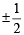Of various possiblities only option (a) is possible.

Test: 35 Year JEE Previous Year Questions: Structure of Atom - Question 9

Consider the ground state of Cr atom (X = 24). The number of electrons with the azimuthal quantum numbers, ℓ = 1 and 2 are, respectively 

Detailed Solution for Test: 35 Year JEE Previous Year Questions: Structure of Atom - Question 9

Electronic configuration of Cr atom (z = 24) = 1s2, 2s2 2p6, 3s2 3p6 3d5, 4s1
when ℓ = 1, p - subshell,
Numbers of electrons = 12
when ℓ = 2, d - subshell,
Numbers of electrons = 5

Test: 35 Year JEE Previous Year Questions: Structure of Atom - Question 10

The wavelength of the radiation emitted, when in a hydrogen atom electron falls from infinity to stationary state 1, would be (Rydberg constant = 1.097×107 m–1) 

Detailed Solution for Test: 35 Year JEE Previous Year Questions: Structure of Atom - Question 10

TIPS/Formulae :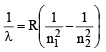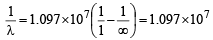λ = 91.15 x 10 -9 m ≈ 91nm

Test: 35 Year JEE Previous Year Questions: Structure of Atom - Question 11

Which one of the followin g sets of ion s represents the collection of isoelectronic species? 

(Atomic nos. : F = 9, Cl = 17, Na = 11, Mg = 12, Al = 13, K = 19, Ca = 20, Sc = 21)

Detailed Solution for Test: 35 Year JEE Previous Year Questions: Structure of Atom - Question 11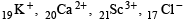each contains 18 electrons.

Test: 35 Year JEE Previous Year Questions: Structure of Atom - Question 12

In a multi-electron atom, which of the following orbitals described by the three quantum members will have the same energy in the absence of magnetic and electric fields? 
(A) n = 1, l = 0, m = 0
(B) n = 2, l = 0, m = 0
(C) n = 2, l = 1, m = 1
(D) n = 3, l = 2, m = 1
(E) n = 3, l = 2, m = 0

Detailed Solution for Test: 35 Year JEE Previous Year Questions: Structure of Atom - Question 12

The energy of an orbital is given by (n + l) in (d) and (c). (n + l) value is (3 + 2) = 5 hence they will have same energy, since there n values are also same.

Test: 35 Year JEE Previous Year Questions: Structure of Atom - Question 13

Of the following sets which one does NOT contain isoelectronic species? 

Detailed Solution for Test: 35 Year JEE Previous Year Questions: Structure of Atom - Question 13

Calculating number of electrons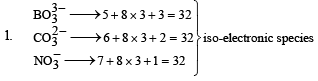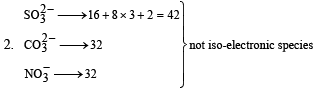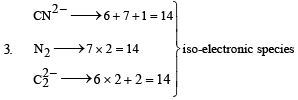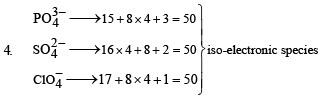Hence the species in option (b) are not isoelectronic.

Test: 35 Year JEE Previous Year Questions: Structure of Atom - Question 14

According to Bohr's theory, the angular momentum of an electron in 5th orbit is 

Detailed Solution for Test: 35 Year JEE Previous Year Questions: Structure of Atom - Question 14

Angular momentum of an electron in nth orbital is given by ,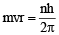For n = 5, we have

Angular momentum of electron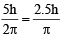Test: 35 Year JEE Previous Year Questions: Structure of Atom - Question 15

Uncertainty in the position of an electron (mass = 9.1 × 10–31 kg) moving with a velocity 300 ms–1, accurate upto 0.001% will be 

(h = 6.63 × 10–34 Js)

Detailed Solution for Test: 35 Year JEE Previous Year Questions: Structure of Atom - Question 15

Given m = 9.1 × 10–31kg , h = 6.6 × 10–34Js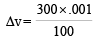= 0.003ms–1

From Heisenberg's uncertainity principle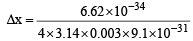= 1.92 x 10-2 m

Test: 35 Year JEE Previous Year Questions: Structure of Atom - Question 16

Which one of the following sets of ions represents a collection of isoelectronic species? 

Detailed Solution for Test: 35 Year JEE Previous Year Questions: Structure of Atom - Question 16

(a)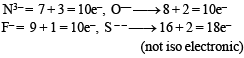(b)  Li+ = 3+1= 4e,  Na+ = 11–1 = 10e,
Mg++  = 12–2=10e
Ca++ = 20 – 2 = 18e–       (not isoelectronic)

(c) K+ = 19 – 1= 18e, Cl =17 + 1  = 18e,
Ca++ = 20 – 2 =18e, Sc3+ = 21–3 = 18e
(isoelectronic)

(d) Ba++ 56 – 2 = 54e,  Sr++ 38–2 = 36e–
K+= 9–1 = 18e,  Ca++= 20–2 = 18e
(not  isoelectronic)

Test: 35 Year JEE Previous Year Questions: Structure of Atom - Question 17

Which of the following sets of quantum numbers represents the highest energy of an atom? 

Detailed Solution for Test: 35 Year JEE Previous Year Questions: Structure of Atom - Question 17

(a) n = 3, ℓ = 0 means 3s-orbital and n + ℓ = 3
(b) n = 3, ℓ = 1 means 3p-orbital n + ℓ = 4
(c) n = 3, ℓ = 2 means 3d-orbital n + ℓ = 5
(d) n = 4, ℓ = 0 means 4s-orbital n + ℓ = 4
Increasing order of energy among these orbitals is 3s < 3p < 4s < 3d
∴ 3d has highest energy..

Test: 35 Year JEE Previous Year Questions: Structure of Atom - Question 18

Which one of the following constitutes a group of the isoelectronic species? 

Detailed Solution for Test: 35 Year JEE Previous Year Questions: Structure of Atom - Question 18

Species having same n umber of el ectr on s ar e isoelectronic calculating the number of electrons in each species given here, we get.
CN (6 + 7 + 1 = 14); N2 (7 + 7 = 14);
O22–(8 + 8 +2 = 18) ; C22– (6 + 6 + 2 = 14);
O2 (8 + 8 + 1 = 17) ; NO+ (7 + 8 – 1 = 14)
CO (6 + 8 = 14) ; NO (7 + 8 = 15)
From the above calculation we find that all the species listed in choice (b) have 14 electrons each so it is the correct answer.

Test: 35 Year JEE Previous Year Questions: Structure of Atom - Question 19

The ionization enthalpy of hydrogen atom is 1.312 × 106 J mol–1. The energy required to excite the electron in the atom from n = 1 to n = 2 is 

Detailed Solution for Test: 35 Year JEE Previous Year Questions: Structure of Atom - Question 19

(ΔE), The energy required to excite an electron in an atom of hydrogen from n = 1 to n = 2 is ΔE  (difference in energy E2 and E1) Values of E2 and E1 are, E1 = – 1.312 × 106 J mol–1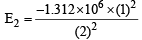= –3.28 × 105 J mol–1

ΔE is given by the relation,

∴ ΔE = E2 – E1  = [–3.28 × 105]– [–1.312 × 106 ] J mol–1

= (–3.28 × 105 + 1.312 × 106) J mol–1

= 9.84 × 105 J mol–1

Thus the correct answer is (d)

Test: 35 Year JEE Previous Year Questions: Structure of Atom - Question 20

Calculate the wavelength (in nanometer) associated with a proton moving at 1.0 × 103 ms –1. (Mass of proton = 1.67 × 10–27 kg and  h = 6.63 × 10–34 Js)

Detailed Solution for Test: 35 Year JEE Previous Year Questions: Structure of Atom - Question 20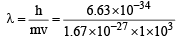= 3.97 × 10–10 meter  = 0.397 nanometer

Test: 35 Year JEE Previous Year Questions: Structure of Atom - Question 21

In an atom, an electron is moving with a speed of 600 m/s with an accuracy of 0.005%. Certainity with which the position of the electron can be located is ( h = 6.6 × 10–34 kg m2s–1, mass of electron, em = 9.1 × 10–31 kg) : 

Detailed Solution for Test: 35 Year JEE Previous Year Questions: Structure of Atom - Question 21

According to Heisenberg uncertainty principle.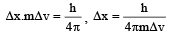Here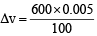= 0.03

So,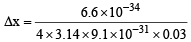= 1.92 × 10–3 meter

Test: 35 Year JEE Previous Year Questions: Structure of Atom - Question 22

The energy required to break one mole of Cl – Cl bonds in Cl2 is 242 kJ mol–1. The longest wavelength of light capable of breaking a single Cl – Cl bond is (c = 3 × 108 ms–1 and NA = 6.02 × 1023 mol–1). 

Detailed Solution for Test: 35 Year JEE Previous Year Questions: Structure of Atom - Question 22

Energy required to break one mole of Cl – Cl bonds in Cl2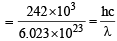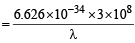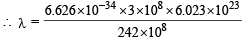= 0.4947 × 10–6 m  = 494.7 nm

Test: 35 Year JEE Previous Year Questions: Structure of Atom - Question 23

Ionisation energy of He+ is 19.6 × 10–18 J atom–1. The energy of the first stationary state (n = 1) of Li2+ is 

Detailed Solution for Test: 35 Year JEE Previous Year Questions: Structure of Atom - Question 23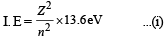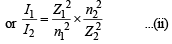Given I1 = – 19.6 × 10–18 , Z1 = 2, n1 = 1 , Z2 = 3 and n2 = 1

Substituting these values in equation (ii).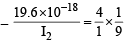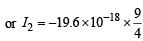= – 4.41 × 10–17 J/atom

Test: 35 Year JEE Previous Year Questions: Structure of Atom - Question 24

A gas a bsor bs a ph oton of 355 n m an d emits at two wavelengths. If one of the emissions is at 680 nm, the other is at:

Detailed Solution for Test: 35 Year JEE Previous Year Questions: Structure of Atom - Question 24

Energy of absorbed photon = Sum of the energies of emitted photon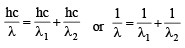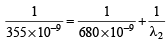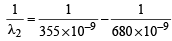= 1.346 x106

or λ2= 1/1.346 × 106 = 743 × 10–9m = 743 nm

Test: 35 Year JEE Previous Year Questions: Structure of Atom - Question 25

The electrons identified by quantum numbers n and l :
(A) n = 4, l = 1
(B) n = 4, l = 0
(C) n = 3, l = 2
(D) n = 3, l = 1
can be placed in order of increasing energy as : 

Detailed Solution for Test: 35 Year JEE Previous Year Questions: Structure of Atom - Question 25

(a) 4 p   (b)  4 s (c) 3 d (d)   3 p

Accroding to Bohr Bury's (n + l) rule, increasing order of energy (D) < (B) < (C) < (A).
Note : If the two orbitals have same value of (n + l) then the orbital with lower value of n will be filled first.

Test: 35 Year JEE Previous Year Questions: Structure of Atom - Question 26

Energy of an electron is given by E = – 2.178 ×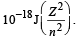Wavelength of light required to excite an electron in an hydrogen atom from level n = 1 to n = 2 will be : (h = 6.62 × 10 –34 Js and c = 3.0 × 108 ms–1) [JEE M 2013]

Detailed Solution for Test: 35 Year JEE Previous Year Questions: Structure of Atom - Question 26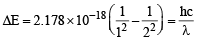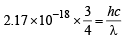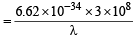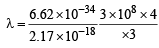= 1.214 × 10–7m

Test: 35 Year JEE Previous Year Questions: Structure of Atom - Question 27

The correct set of four quantum numbers for the valence electrons of rubidium atom (Z = 37) is: [JEE M 2014]

Detailed Solution for Test: 35 Year JEE Previous Year Questions: Structure of Atom - Question 27

The electronic configuration of Rubidium (Rb = 37) is

1s22s2 2 p6 3s23 p6 3d10 4s2 4 p6 5s1
Since last electron enters in 5s orbital Hence n = 5, l = 0, m = 0, s =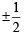Test: 35 Year JEE Previous Year Questions: Structure of Atom - Question 28

Which of the following is the energy of a possible excited state of hydrogen ? [JEE M 2015]

Detailed Solution for Test: 35 Year JEE Previous Year Questions: Structure of Atom - Question 28

Total energy =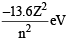where n = 2, 3, 4 ....
Putting n = 2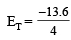= -3.4eV

Test: 35 Year JEE Previous Year Questions: Structure of Atom - Question 29

A stream of electrons from a heated filaments was passed two charged plates kept at a potential difference V esu. If e and m are charge and mass of an electron, respectively, then the value of h/λ (where λ is wavelength associated with electron wave) is given by: [JEE M 2016]

Detailed Solution for Test: 35 Year JEE Previous Year Questions: Structure of Atom - Question 29

As electron of charge 'e' is passed through 'V' volt, kinetic energy of electron will be eV

Wavelength of electron wave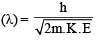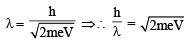## Chemistry for JEE

322 videos|586 docs|368 tests
 Use Code STAYHOME200 and get INR 200 additional OFF Use Coupon Code
Information about Test: 35 Year JEE Previous Year Questions: Structure of Atom Page
In this test you can find the Exam questions for Test: 35 Year JEE Previous Year Questions: Structure of Atom solved & explained in the simplest way possible. Besides giving Questions and answers for Test: 35 Year JEE Previous Year Questions: Structure of Atom, EduRev gives you an ample number of Online tests for practice

## Chemistry for JEE

322 videos|586 docs|368 tests